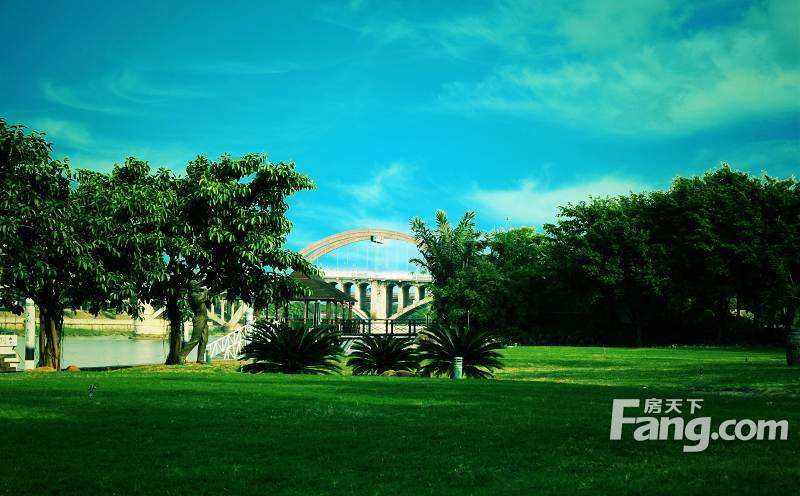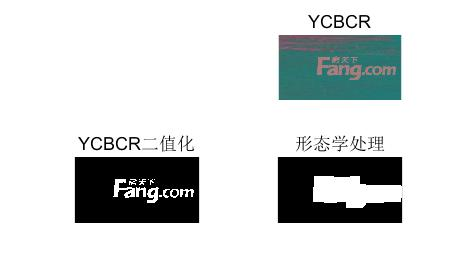• 例子是用来提取叶片形状参数的，如果感兴趣可以拿去参考，修改。。。
• matlab 任意轮廓外接矩形代码及其实现； clc;clear;close all; I=imread('00121.bmp'); I=rgb2gray(I); subplot(3,3,1);imshow(I); % M=im2double(I);%将unit8转成double型 subplot(3,3,2),imhist(I); M1=...
matlab 任意轮廓外接矩形代码及其实现；

clc;clear;close all;
I=rgb2gray(I);
subplot(3,3,1);imshow(I);
% M=im2double(I);%将unit8转成double型
subplot(3,3,2),imhist(I);
M1=im2bw(I,25/255);%二值图level取值0~1.
subplot(3,3,3);imshow(M1);
BW1=1-M1;%取反操作
subplot(3,3,4);imshow(BW1);
BW2=bwareaopen(BW1,100000,4);%形态学操作，删除二值图像BW中面积小于10000的对象,4邻域
subplot(3,3,5);imshow(BW2);
BW3=1-BW2;
subplot(3,3,6);imshow(BW3);
BW=bwareaopen(BW3,90000);%形态学操作，删除二值图像BW中面积小于10000的对象,8邻域
subplot(3,3,7);imshow(BW);xlabel('除去背景后的完整二值图');
se=strel('disk',4);
% se1=strel('disk',4);
J=imerode(BW,se);
% J1=imerode(BW,se1);
J=BW-J;
subplot(3,3,8);imshow(J);xlabel('4直径');
% subplot(3,3,8);imshow(J1);xlabel('4直径');
J=edge(BW,'canny',0.3,2);
subplot(3,3,9);imshow(J);

整理好的下面。

clc;clear;close all;
I=rgb2gray(I);
subplot(3,3,1);imshow(I);
%%
% M=im2double(I);%将unit8转成double型
subplot(3,3,2),imhist(I);
M1=im2bw(I,25/255);%二值图level取值0~1.
subplot(3,3,3);imshow(M1);
BW1=1-M1;%取反操作
subplot(3,3,4);imshow(BW1);
BW2=bwareaopen(BW1,100000,4);%形态学操作，删除二值图像BW中面积小于10000的对象,4邻域
subplot(3,3,5);imshow(BW2);
BW3=1-BW2;
subplot(3,3,6);imshow(BW3);
BW=bwareaopen(BW3,90000);%形态学操作，删除二值图像BW中面积小于10000的对象,8邻域
subplot(3,3,7);imshow(BW);xlabel('除去背景后的完整二值图');
%%
se=strel('disk',4);
% se1=strel('disk',4);
J=imerode(BW,se);
% J1=imerode(BW,se1);
J=BW-J;
J=bwmorph(J,'clean',10);%清除杂点，孤立点
J=bwmorph(J,'spur',Inf);%去除小分支，去毛刺
subplot(3,3,8);imshow(J);xlabel('4直径');
%%
[x,y]=find(J==1);%%返回的是行列像素不为0的点的纵坐标，横坐标
xmi=min(x(:));
xma=max(x(:));
ymi=min(y(:));
yma=max(y(:));
dx=xma-xmi; %%矩形宽
dy=yma-ymi; %%矩形长
rectangle('Position',[ ymi xmi dy dx],'EdgeColor','g', 'LineWidth',1);
s=dy*dx;
% [BW,thresh] = edge(BW,'canny');
Jj=edge(BW,'canny',[0.0063,0.0156],1.4);%灵敏度，高斯滤波标准差1.4
j=bwmorph(Jj,'clean',10);
subplot(3,3,9);imshow(j);

%%
L=bwlabel(j,8);%标记连通区域，选择8邻域则可以标记成功，
stats=regionprops(L,'BoundingBox');
temp = stats.BoundingBox;
rectangle('position',temp,'edgecolor','r');

clc;clear;close all;
I=rgb2gray(I);
subplot(3,3,1);imshow(I);
% M=im2double(I);%将unit8转成double型
subplot(3,3,2),imhist(I);
M1=im2bw(I,25/255);%二值图level取值0~1.
subplot(3,3,3);imshow(M1);
BW1=1-M1;%取反操作
subplot(3,3,4);imshow(BW1);
BW2=bwareaopen(BW1,100000,4);%形态学操作，删除二值图像BW中面积小于10000的对象,4邻域
subplot(3,3,5);imshow(BW2);
BW3=1-BW2;
subplot(3,3,6);imshow(BW3);
BW=bwareaopen(BW3,90000);%形态学操作，删除二值图像BW中面积小于10000的对象,8邻域
subplot(3,3,7);imshow(BW);xlabel('除去背景后的完整二值图');
se=strel('disk',4);
% se1=strel('disk',4);
J=imerode(BW,se);
% J1=imerode(BW,se1);
J=BW-J;
J=bwmorph(J,'clean',10);
subplot(3,3,8);imshow(J);xlabel('4直径');
[x,y]=find(J==1);%%返回的是行列像素不为0的点的纵坐标，横坐标
xmi=min(x(:));
xma=max(x(:));
ymi=min(y(:));
yma=max(y(:));
dx=xma-xmi; %%矩形宽
dy=yma-ymi; %%矩形长
rectangle('Position',[ ymi xmi dy dx],'EdgeColor','g', 'LineWidth',1);
s=dy*dx;
[BW,thresh] = edge(BW,'canny');
Jj=edge(BW,'canny',[0.0063,0.0156],1.4);%灵敏度，高斯滤波标准差1.4
j=bwmorph(Jj,'clean',10);
j=bwmorph(Jj,'spur',10);%去除小分支，去毛刺
subplot(3,3,9);imshow(j);

%%
L=bwlabel(j,8);
stats=regionprops(L,'BoundingBox');
temp = stats.BoundingBox;
rectangle('position',temp,'edgecolor','r');


展开全文• 想用MATLAB做一个提取运动目标的功能，如图![图片说明](https://img-ask.csdn.net/upload/201911/23/1574496675_713294.png) 代码如下但是现象极其糟糕 不知道错误处在哪里 请各位大神指教  fileName = '...神经网络
• 提取目标是右下角的水印。 首先放一个手动截图的matlab代码，用它可以实现截取图片的一部分并保存。 %从图片中选取矩形框区域 P_frist = imread('p1.jpg'); [A,rect] = imcrop(P_frist); figure(...
首先感谢博客 这篇博客中提到了一种基于颜色的人脸识别，很好的例子！ 本文主要是按他的思路来的。 目标是实现图片水印的目标提取。 原图如下所示：提取目标是右下角的水印。
首先放一个手动截图的matlab代码，用它可以实现截取图片的一部分并保存。
%从图片中选取矩形框区域
[A,rect] = imcrop(P_frist);
figure(3);
imshow(A);
rect;
%截取矩形区域图像并保存
P_cut = imcrop(P_frist,rect);

这里可以手动获得感兴趣的矩形截图。
上面提到了博客中，将RGB转换到YCBCR的色彩空间，再进行处理。 这里简单说明 Y：明亮度。“亮度”是透过RGB输入信号来建立的，方法是将RGB信号的特定部分叠加到一起。 CB：是RGB图像的蓝色部分与RGB亮度值之间的差异。 CR：是RGB图像的红色部分与RGB亮度值之间的差异。 RGB和YCbCr各分量的值的范围均为0-255。
转换公式： Y = 0.257R+0.564G+0.098B+16 Cb = -0.148R-0.291G+0.439B+128 Cr = 0.439R-0.368G-0.071*B+128
R = 1.164*(Y-16)+1.596*(Cr-128) G = 1.164*(Y-16)-0.392*(Cb-128)-0.813*(Cr-128) B = 1.164*(Y-16)+2.017*(Cb-128)
转换完成后，通过选择各个通达的门限值实现二值化，门限值可以更新，兴趣区域的具体数值进行确定。
%matrix（用各个通道的阈值对其进行二值化处理）
Y_MIN = 140;  Y_MAX = 200;
Cb_MIN = 110;   Cb_MAX = 140;
Cr_MIN = 110;   Cr_MAX = 130;
threshold=roicolor(YCBCR(:,:,1),Y_MIN,Y_MAX)&roicolor(YCBCR(:,:,2),Cb_MIN,Cb_MAX)&roicolor(YCBCR(:,:,3),Cr_MIN,Cr_MAX);
subplot(2,2,3),imshow(threshold),title('YCBCR二值化'),

这里的门限值为水印的范围。
然后进行形态学处理，包括 imerode（腐蚀），imdilate（膨胀），imfill（孔洞填充） 这样就获得了兴趣区域的提取。 如图：到这基本完成任务了，提取目标玩以后就是要对目标进行处理。 对于水印的处理一般为扣除后进行添补，也就是常说的 inpaint。 这里提供一个matlab，进行inpaint的优秀代码，之后有时间会把它和本例结合。 这里就简单的给个例子。
%膜P_mask
%
%窗口尺寸为N
N=3;
for i=2:m-1
for j=2:n-1
%过窗
for size_i=1:N
for size_j=1:N
P_cut(i+size_i-2,j+size_j-2)=P_cut(i,j);
end
end
end

end
end
figure(2);
imshow(P_cut),title('tu');

实现的是用3*3的窗口对兴趣区域进行处理，可以根据自己的算法进行改进。
如有不对的地方欢迎指正，共同学习。
展开全文数字图像处理
• 为对一幅图像中目标区域提取颜色，我做了如下处理，读图像，对目标区域进行裁剪，对目标区域进行RGB提取，对多个目标区域进行RGB均值绘制，寻找规律，以便可以更好的做目标提取。 function [ obj_rgbmean ] = ...
为对一幅图像中目标区域提取颜色，我做了如下处理，读图像，对目标区域进行裁剪，对目标区域进行RGB提取，对多个目标区域进行RGB均值绘制，寻找规律，以便可以更好的做目标提取。

function [ obj_rgbmean ] = getcolors( Image, n) %UNTITLED3 此处显示有关此函数的摘要 %   此处显示详细说明 %Image 待检测图像 %n 截取图片的次数 %obj_rgbmean目标图像rjb均值矩阵 obj_rgbmean=[];%用来存放目标图像rjb均值矩阵 nR = []; %用来存放矩形区域RGB均值的数组 nG = []; nB = []; figure for i=1:n imshow(Image); h = imrect;%鼠标变成十字，用来选取感兴趣区域 pos = getPosition(h); %pos有四个值，分别是矩形框的左下角点的坐标 x y 和 框的 宽度和高度 imag = imcrop(Image, pos);%imcrop(I,[a b c d]);利用裁剪函数裁剪图像 imshow(imag);
image_R=imag(:,:,1); %构建RGB三个矩阵 image_G=imag(:,:,2); image_B=imag(:,:,3);
R=mean(image_R(:)); %计算RGB三个矩阵的均值 G=mean(image_G(:)); B=mean(image_B(:)); nR(i)=R;%将每个图像的RGB矩阵均值存入nr、ng、nb数组中 nG(i)=G; nB(i)=B; end obj_rgbmean=cat(1,nR,nG,nB); figure plot(1:n,nR,'r', 1:n,nG,'g', 1:n, nB,'b');%绘制所有分帧区域内图像的RGB矩阵均值折线图
%%RGB曲线图注释说明
xlabel('目标数');  %曲线图注释说明 ylabel('均值'); title('区域RGB均值曲线图'); legend('R', 'G', 'B'); end
展开全文机器学习
• 本程序针对二值化后分割图像进行感兴趣区域提取，对研究图像分割区域提取有一定的参考价值。 clc clear close all I= imread('gend.bmp'); figure(1); imshow(I); I=im2bw(I); [L, num] = bwlabel(I); STATS1=...
本程序针对二值化后的分割图像进行感兴趣区域提取，对研究图像分割区域提取有一定的参考价值。
clc clear
close all
figure(1);
imshow(I);
I=im2bw(I);
[L, num] = bwlabel(I);
STATS1=regionprops(L,'Perimeter');
ahe=size(STATS1);
figure(2);
imshow(I);
m1=ahe(1,1);
m=zeros(2,m1);
for i=1:m1
% 计算目标区域中心，用于显示编号的位置
[p,q]=find(L==i);
temp=[p,q];
[x,y]=size(temp);
m(1,i)=sum(p)/x;
m(2,i)=sum(q)/x;
end
for i=1:m1
figure(2);
text(m(2,i),m(1,i),int2str(i),'color','red')
end
L(L~=3&L~=2)=0;        %%这边进行区域的选择，例如只保留2、3.
figure(3);

imshow(L);
`
展开全文• Matlab 利用鼠标选择目标区域 ！总是遗忘一些知识，翻找以前的东西很麻烦，就当备忘录了！ 使用imrect()函数 % ------------------ % Matlab手动鼠标截图，获取兴趣区域 % ------------------ clc; clear; close ...
• Matlab提取RGB颜色分量： 我们知道，一张RGB图像，对应着一个M xN x3 的三维图像。这里的3代表着R,G,B三个分量。其数据类型为uint8类型。数值大小在[0,255]之间。 例如：我们在Matlab中读入本次测试的图像。I=...阈值分割 图像处理
• 基于matlab工具箱提取图像中的多目标特征（代码如下）： 代码前面部分为提取图像的边界信息，调用了后面的遍历函数Pixel_Search，函数实现方法见后~ %%ROI Testing close all; clear all; clc; I=imread('...
• 对于图像感兴趣区域（ROI）的提取，一般从来两个方面着手解决：一是利用图像分割技术提取ROI；二是从人眼得视觉特征出发，通过模拟人眼得视觉特点，寻找特定得视觉敏感区域，并将这些视觉敏感区域排序作为ROI。 本文...
• ## 图像处理之Matlab特征提取和表达

万次阅读 多人点赞 2017-08-07 17:36:14
介绍图像处理之特征提取和表达，使用bwboundaries函数获取边界，使用regionprops函数统计特征函数 图像处理 图形 计算机视觉
• 原图如下所示：提取目标是右下角的水印。首先放一个手动截图的matlab代码，用它可以实现截取图片的一部分并保存。%从图片中选取矩形框区域P_frist = imread('p1.jpg');[A,rect] = imcrop(P_frist);figure(3);imshow...
• 基于MATLAB的动态前景目标特征提取与运动跟踪 摘 要：动态前景目标识别和提取是计算机视觉领域的重要内容。对动态图像进行前景目标提取与运动跟踪，通过改进高斯混合背景模型，提出一种基于自适应特征加权的前景目标...算法 计算机视觉 机器学习 人工智能 深度学习
• 本发明属于图像处理技术，具体涉及一种基于形态学的目标特征提取方法。背景技术：特征提取是图像识别的关键技术，图像的特征有颜色特征，纹理特征，几何特征等等。目标的几何特征是图像识别中常用的特征。通常目标...
• ﻿﻿ 在工程设计中，常常需要查询datasheet并根据其...接下来我们就介绍一种利用MATLAB提取曲线数据的方法，为下一步进行曲线拟合做准备。 首先，我们任意选取一个我们希望提取数据的曲线，如图1所示。目标是通过图片 数据
• 利用掩膜图像来获取图像的任意区域，该程序可以直接运行。掩膜图像
• % 获取感兴趣区域，并进行提取和标记 % 编写时间：2018年3月28日22:40:46 编写人：gq close all; clear all; clc; srcImage = imread('grh.bmp'); whos, % 显示原始图像 figure('name', '前期处理'); subplot...
• ## matlab特定颜色提取

万次阅读 多人点赞 2019-02-28 10:02:33
matlab特定颜色提取 一、理论基础 在电脑中，RGB的所谓“多少”就是指亮度，并使用整数来表示。通常情况下，RGB各有256级亮度，用数字表示为从0、1、2…直到255。注意虽然数字最高是255，但0也是数值之一，因此共256...
• 拍一幅含多个人体目标的图像，利用外接盒、围盒和凸包对每个人体目标区域进行表达，并提取其骨架 main clc clear close all %% 原图 figure(1) img=imread('人物剪影.jpg'); imshow(img); title('原图'); %% 二值图 ...
• 本文介绍了一种基于MATLAB的简易的从视频播放的帧图像中找出目标图像，并进行视频跟踪的实现方法。通过对图像进行阈值处理（图像分割），再对分割后的图像求取形心，以对目标图像进行定位，并最后找...dbcp webgl 信号处理 dwr 黑盒测试
• 选择你感兴趣的区域，截取图片，然后下一步对你感兴趣的区域继续进行操作
• 本文提出了一种基于累积差分和数学形态学处理的运动区域提取算法。在时域窗口内，首先对图象进行降级处理得到灰度带图象，对灰度带差分图象累积并进行数学形态学处理得到运动目标的轨迹模版，将轨迹模版与当前帧差分...算法 image algorithm 测试
• 1 绪论课题研究背景及意义运动目标检测是图像处理与计算机视觉的一个分支，在理论和实践上都有重大意义， 长久以来一直被国内外学者所关注。在实际中，视频监控利用摄像机对某一特定区域进行 监视，是一个细致和连续...算法 编程语言 计算机视觉 机器学习 人工智能图像处理
• 基于matlab工具箱提取图像中的多目标特征（代码如下）： 代码前面部分为提取图像的边界信息，调用了后面的遍历函数Pixel_Search，函数实现方法见后~ %%ROI Testing close all; clear all; clc; I=imread('...
• 1　绪论1.1　课题研究背景及意义运动目标检测是图像处理与计算机视觉的一个分支，在理论和实践上都有重大意义，长久以来一直被国内外学者所关注。在实际中，视频监控利用摄像机对某一特定区域进行监视，是一个细致和...算法 编程语言 计算机视觉 机器学习 人工智能
• 图像处理之目标检测目标检测的基本思路遇到的问题鹿人鹰花狗总结附录 ...对于彩色图像想要提取某一特定颜色阈值比较难选取，图像中目标的颜色不是唯一的，有彩色和黑色和白色，可以通过将rgb空间转换为ycbc边缘检测 计算机视觉 图像识别
• MATLAB代码： %边界跟踪 bwtraceboundary 函数 clc I = imread('../deep.jpg'); figure imshow(I); title('原始图像'); I1 = rgb2gray(I); %彩图转换成灰度图像 %使用阈值 目的是将图像转换为二进制图像 threshold......

# matlab提取目标区域matlab 订阅